# Electronics and Communication Engineering - Exam Questions Papers

11.

The z-transform of a signal is given by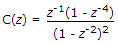. Its final value is :

 A. 1/4 B. zero C. 1 D. infinity

Explanation:

Final value is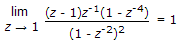.

12.

The residue of the function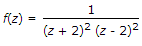at z = 2 is

 A.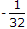B.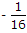C.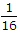D.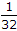Explanation:

Residue at z = 2.

13.

The number of variables in a function is odd and the number of minterms with even number of 0's are same as the number of minterms with odd number at 1's, the expression formed can be represented in __________ as well as __________ form.

 A. Ex - OR, NOR B. Equivalence, Ex - OR C. AND, Coincidence D. OR, Ex - OR

Explanation:

With odd number of variables (n)

Let n = 3, by using k map

A (B ⊕ C) + A(BC + B C)

= A ⊕ D

D = B⊕C

D = B⊕C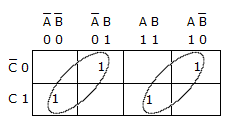This can be represented by EX-NOR of EX-OR expressions.

EX-NOR means equivalence.

14.

In a number system with radix r, the decimal value of 110 is equal to 4r. The decimal values of 111 and r respective are equal to

 A. 20, 5 B. 16, 4 C. 13, 3 D. 8, 2

Explanation:

(110)r = 4r

r2 + r = 4r

r(r - 3) = 0 ⇒ r ≠ 0, r = 3

(lll)r = 32 + 3 + 1 = 13.

15.

The electric field of uniform plane wave is given by E = 20 sin (2 p x 108t- pz)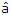x + 20 cos (2 p x 108t - pz)y. Find phase velocity

 A. 2 x 108 m/s B. p x 108 m/s C. 2p x 108 m/s D. none of these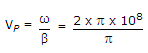= 2 x 108 m/s.